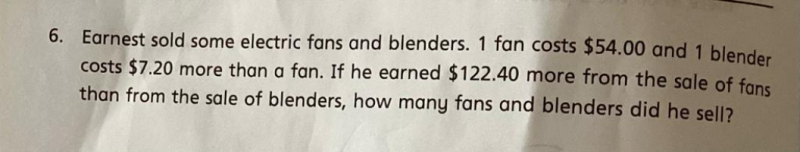# QuestionNumber of fans sold = 122.40 / 7.2  = 17    (Difference in amount attributed to their price difference)

Total sales for fans = 17 x 54 = 918

Total sales for blenders = 918 – 122.40 = 795.60

Number of blenders sold = 795.60/ 61.20  = 13

Hence 17 fans and 13 blenders sold.

Please click ” Accept Answer” if you accept the answer.

0 Replies 1 Like ✔Accepted Answer